基于两种回归的指数追踪模型以及实证分析 Index Tracking Model Based on Two Regressions and Empirical Analysis

• 全文下载: PDF(3481KB)    PP.60-78   DOI: 10.12677/SA.2019.81008
• 下载量: 152  浏览量: 328

Index tracking is a method of tracking the trend of a certain market index with a small number of constituent stocks. It is one of the negative portfolio management strategies and has developed rapidly in China in recent years. In this paper, three sample stocks are selected by three stock selection methods, and an exponential tracking model based on linear regression is constructed. In order to avoid the linear regression coefficient being affected by extreme values, this paper also establishes index tracking based on quantile regression model. Specifically, this paper selects the SSE 50 Index as the target index, and establishes two index tracking models by giving the constraints of establishing the index tracking model, and finally passes the maximum weight stock selection method, the maximum market value selection method and the maximum correlation. The coefficient stock selection method selects stocks of the SSE 50 index, selects the first 15 stocks of each stock picking method as the sample stock space, and finally assigns weights to the selected constituent stocks by fitting the real data.

1. 引言

2. 变量介绍

${\epsilon }_{i}$ ，指数追踪组合中第i只股票的最小投资比例；

${\delta }_{i}$ ，指数追踪组合中第i只股票的最大投资比例；

${X}_{i}$ ，当前追踪组合中第i只股票的持有数量，若新建追踪组合，则 ${X}_{i}=0$

${V}_{it}$ ，第i只股票在t时刻的价格；

${I}_{t}$ ，所追踪的市场指数在t时刻的价格；

${r}_{it}$ ，所构建的追踪组合在t时刻的收益率，本文中 ${r}_{it}=\mathrm{ln}\frac{{V}_{it}}{{V}_{i,t-1}}$

${R}_{t}$ ，所追踪的市场指数在t时刻的收益率，本文中 ${R}_{t}=\mathrm{ln}\frac{{I}_{t}}{{I}_{t-1}}$

C，构建指数追踪的总资本，总资本等于指数追踪的总价格和交易费之和；

${f}_{i}^{s}$ ，卖出第i只股票所需要缴纳的经手费和证管费的比率；

${f}_{i}^{b}$ ，买入第i只股票所需要缴纳的经手费和证管费的比率；

${h}_{i}^{s}$ ，卖出第i只股票所需要缴纳的过户费的比率；

${h}_{i}^{b}$ ，买入第i只股票所需要缴纳的过户费的比率；

${M}_{i}^{s}$ ，在t时刻第i只股票能够卖出的最大数量；

${M}_{i}^{b}$ ，在t时刻第i只股票能够买入的最大数量；

$\gamma$ ，总交易费用在总资本中的最大占比，且 $0<\gamma <1$

${x}_{i}$ ，新的指数追踪组合中第i只股票的持有数量；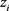，是一个0~1变量， ${z}_{i}=1$ 表示在新的指数追踪中有第i只股票， ${z}_{i}=0$ 表示在新的指数追踪中没有第i只股票；

${a}_{i}^{s}$ ，是一个0~1变量， ${\alpha }_{i}^{s}=1$ 表示卖出第i只股票， ${\alpha }_{i}^{s}=0$ 表示没卖出第i只股票；

${a}_{i}^{b}$ ，是一个0~1变量，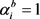表示买入第i只股票， ${\alpha }_{i}^{s}=0$ 表示没买入第i只股票；

 ，表示第i只股票的卖出数量， ${y}_{i}^{s}\ge 0$

${p}_{i}^{b}$ ，表示第i只股票的买入数量， ${y}_{i}^{b}\ge 0$

1) 交易有成本。

2) 股票不能够卖空，即 ${x}_{i}\ge 0$

3) 由于中国股市的股票是以一手(100股)为单位买卖的，因此 ${x}_{i}$ 是以百为单位的一个数。

3. 约束条件

${\sum }_{i=1}^{N}{z}_{i}=K$ (3.1)

${\epsilon }_{i}{z}_{i}\le \frac{{x}_{i}{V}_{it}}{C}\le {\delta }_{i}{z}_{i},\text{\hspace{0.17em}}\text{\hspace{0.17em}}\text{\hspace{0.17em}}i=1,\cdots ,N$ (3.2)

${x}_{i}={X}_{i}+{y}_{i}^{b}-{y}_{i}^{s},\text{\hspace{0.17em}}\text{\hspace{0.17em}}\text{\hspace{0.17em}}i=1,\cdots ,N$ (3.3)

${a}_{i}^{s}+{a}_{i}^{b}\le 1,\text{\hspace{0.17em}}\text{\hspace{0.17em}}\text{\hspace{0.17em}}i=1,\cdots ,N$ (3.4)

${p}_{i}^{s}\le \mathrm{min}\left[{M}_{i}^{s},{x}_{i}\right]{a}_{i}^{s},\text{\hspace{0.17em}}\text{\hspace{0.17em}}\text{\hspace{0.17em}}i=1,\cdots ,N$ (3.5)

${p}_{i}^{b}\le {M}_{i}^{b}{a}_{i}^{b},\text{\hspace{0.17em}}\text{\hspace{0.17em}}\text{\hspace{0.17em}}i=1,\cdots ,N$ (3.6)

${h}_{i}^{s}=⌈\frac{{y}_{i}^{s}}{1000}⌉\ast 1,\text{\hspace{0.17em}}\text{\hspace{0.17em}}\text{\hspace{0.17em}}i=1,\cdots ,N$ (3.7)

${h}_{i}^{b}=⌈\frac{{y}_{i}^{b}}{1000}⌉\ast 1,\text{\hspace{0.17em}}\text{\hspace{0.17em}}\text{\hspace{0.17em}}i=1,\cdots ,N$ (3.8)

${\sum }_{i=1}^{N}{V}_{iT}{x}_{i}=C-{\sum }_{i=1}^{N}\left({f}_{i}^{s}{V}_{iT}{p}_{i}^{s}+{f}_{i}^{b}{V}_{iT}{p}_{i}^{b}+{h}_{i}^{s}+{h}_{i}^{b}\right)$ (3.9)

${\sum }_{i=1}^{N}\left({f}_{i}^{s}{V}_{iT}{p}_{i}^{s}+{f}_{i}^{b}{V}_{iT}{p}_{i}^{b}+{h}_{i}^{s}{a}_{i}^{s}+{h}_{i}^{b}{a}_{i}^{b}\right)\le \gamma C$ (3.10)

${p}_{i}^{s},{p}_{i}^{b},{x}_{i}\ge 0,\text{\hspace{0.17em}}\text{\hspace{0.17em}}\text{\hspace{0.17em}}i=1,\cdots ,N$ (3.11)

${a}_{i}^{s},{a}_{i}^{b},{z}_{i}\in \left[0,1\right],\text{\hspace{0.17em}}\text{\hspace{0.17em}}\text{\hspace{0.17em}}i=1,\cdots ,N$ (3.12)

(3.1)式是确保在新的指数追踪组合中只有K只股票；(3.2)式是确保如果第i只股票在新的追踪组合中(即 ${z}_{i}=1$ )，那么它的持有量满足一个上下限，如果第i只股票不在新的追踪组合中(即 ${z}_{i}=0$ )，那么它的持有量为0；(3.3)式表示经过重新调整之后第i只股票在新的追踪组合中的持有量；(3.4)式是确保在重新调整组合的过程中，第i只股票不会同时发生买入和卖出；(3.5)式是确保第i只股票的卖出量不超过 ${M}_{i}^{s}$${x}_{i}$ 的最小值，如果没有卖出，那么 ${p}_{i}^{s}$ 为0；(3.6)式确保第i只股票的买入量不超过 ${M}_{i}^{b}$ ，如果没有买入，那么 ${p}_{i}^{b}$ 为0；(3.7)式和(3.8)式则分别定义了第i只股票卖出和买入时所需要缴纳的过户费，由于上海证券中以6为首位数的股票都需要缴纳过户费，且每一千股缴1元，不足一千股按一千股计算；(3.9)式是一个平衡约束，即新的追踪组合的总价值等于总投资金额(当前追踪组合在T时刻的总价值加上现金变化，即C)减去总的交易费用；(3.10)式是对总交易费用设定一个适当的界限；(3.11)式定义连续变量 ${y}_{i}^{s},{y}_{i}^{b},{x}_{i}$ 非负，(3.12)式定义 ${a}_{i}^{s},{a}_{i}^{b},{z}_{i}$ 为0~1变量。

① 公式(3.1)中K关系到追踪组合中股票种类数量；

② 公式(3.2)中 ${\epsilon }_{i}$${\delta }_{i}$ 关系到第i只股票的最小和最大投资比例；

③ 公式(3.5)和公式(3.6)中 ${M}_{i}^{s}$${M}_{i}^{b}$ 关系到第i只股票能够卖出和买入的最大数量；

④ 公式(3.10)中 $\gamma$ 关系到总交易费用在总投资额中的最大占比。

4. 非线性转换

1。假设所构建的追踪组合中的第i只股票在t时刻的所占权重为 ${W}_{it}$ ，其中  ，且 ${\sum }_{i=1}^{N}{W}_{it}=1,i=1,\cdots ,N$ ，那么追踪组合在t时刻的总收益为 ${\sum }_{i=1}^{N}{W}_{it}{r}_{it}$

${w}_{i}=\frac{{x}_{i}{V}_{iT}}{{\sum }_{j=1}^{N}{x}_{j}{V}_{jT}}=\frac{{x}_{i}{V}_{iT}}{C-{\sum }_{i=1}^{N}\left({f}_{i}^{s}{V}_{iT}{p}_{i}^{s}+{f}_{i}^{b}{V}_{iT}{p}_{i}^{b}+{h}_{i}^{s}+{h}_{i}^{b}\right)}$ (4.1)

${w}_{i}=\frac{{x}_{i}{V}_{iT}}{C-{\sum }_{i=1}^{N}\left({f}_{i}^{s}{V}_{iT}{p}_{i}^{s}+{f}_{i}^{b}{V}_{iT}{p}_{i}^{b}+{h}_{i}^{s}+{h}_{i}^{b}\right)}\le \frac{{x}_{i}{V}_{iT}}{C-\gamma C}$ (4.2)

${w}_{i}=\frac{{x}_{i}{V}_{iT}}{C-\gamma C},\text{\hspace{0.17em}}\text{\hspace{0.17em}}\text{\hspace{0.17em}}i=1,\cdots ,N$ (4.3)

5. 回归模型建立

5.1. 基于线性回归的指数追踪

${r}_{it}={\alpha }_{i}+{\beta }_{i}{R}_{t}+{\epsilon }_{i}$

${y}_{t}={w}_{1}{r}_{1t}+{w}_{2}{r}_{2t}+\cdots +{w}_{N}{r}_{Nt}={\sum }_{i=1}^{N}{w}_{i}{\alpha }_{i}+{\sum }_{i=1}^{N}{w}_{i}{\beta }_{i}{R}_{t}$ (5.1)

${\sum }_{i=1}^{N}{w}_{i}{\alpha }_{i}=\alpha$${\sum }_{i=1}^{N}{w}_{i}{\beta }_{i}=\beta$ ，那么公式(5.1)可以简写成

${y}_{t}=\alpha +\beta {R}_{t}$ (5.2)

${\sum }_{i=1}^{N}{w}_{i}{\stackrel{^}{\alpha }}_{i}=\stackrel{^}{\alpha }$${\sum }_{i=1}^{N}{w}_{i}{\stackrel{^}{\beta }}_{i}=\stackrel{^}{\beta }$ 。现在目标是使得 $\stackrel{^}{\alpha }=0$$\stackrel{^}{\beta }=1$ ，下面我们将介绍一种方法来尽可能达到

${\mathrm{min}}_{w}{\lambda }_{1}|\stackrel{^}{\alpha }-0|+{\lambda }_{2}|\stackrel{^}{\beta }-1|$ (5.3)

5.2. 基于分位数回归的指数追踪

5.2.1. 分位数回归参数估计

${\mathrm{min}}_{{\alpha }_{q},{\beta }_{q}}{\sum }_{i=1}^{n}\left(q-{I}_{{Y}_{i}\le {\alpha }_{q}+{\beta }_{q}{X}_{i}}\right)\left({Y}_{i}-\left({\alpha }_{q}+{\beta }_{q}{X}_{i}\right)\right)$ (5.4)

$\begin{array}{l}\left(q-{I}_{{Y}_{i}\le {\alpha }_{q}+{\beta }_{q}{X}_{i}}\right)\left({Y}_{i}-\left({\alpha }_{q}+{\beta }_{q}{X}_{i}\right)\right)\\ =\left(q-{I}_{{Y}_{i}\le {\alpha }_{q}+{\beta }_{q}{X}_{i}}\right){u}_{i}=\left\{\begin{array}{l}\left(q-1\right){u}_{i}\text{\hspace{0.17em}}\text{\hspace{0.17em}}\text{\hspace{0.17em}}\text{\hspace{0.17em}}如果{Y}_{i}\le {\alpha }_{q}+{\beta }_{q}{X}_{i}\\ q{u}_{i}\text{\hspace{0.17em}}\text{\hspace{0.17em}}\text{\hspace{0.17em}}\text{\hspace{0.17em}}\text{\hspace{0.17em}}\text{\hspace{0.17em}}\text{\hspace{0.17em}}\text{\hspace{0.17em}}\text{\hspace{0.17em}}\text{\hspace{0.17em}}\text{\hspace{0.17em}}\text{\hspace{0.17em}}\text{\hspace{0.17em}}\text{\hspace{0.17em}}\text{\hspace{0.17em}}\text{\hspace{0.17em}}\text{\hspace{0.17em}}\text{\hspace{0.17em}}\text{\hspace{0.17em}}其他\end{array}\end{array}$

${\mathrm{min}}_{{\alpha }_{q},{\beta }_{q}}q\left({\sum }_{i=1,{u}_{i}\ge 0}^{n}|{u}_{i}|\right)+\left(1-q\right)\left({\sum }_{i=1,{u}_{i}<0}^{n}|{u}_{i}|\right)$ (5.5)

${\mathrm{min}}_{{\alpha }_{q},{\beta }_{q}}q\left({\sum }_{i=1}^{n}{u}_{i}^{+}\right)+\left(1-q\right)\left({\sum }_{i=1}^{n}{u}_{i}^{-}\right)$ (5.6)

$\text{s}.\text{t}.\text{\hspace{0.17em}}\text{\hspace{0.17em}}{u}_{i}^{+}\ge {Y}_{i}-\left({\alpha }_{q}+{\beta }_{q}{X}_{i}\right),\text{\hspace{0.17em}}\text{\hspace{0.17em}}i=1,\cdots ,n$ (5.7)

${u}_{i}^{-}\ge -\left[{Y}_{i}-\left({\alpha }_{q}+{\beta }_{q}{X}_{i}\right)\right],\text{\hspace{0.17em}}\text{\hspace{0.17em}}i=1,\cdots ,n$ (5.8)

${u}_{i}^{+},{u}_{i}^{-}\ge 0$ (5.9)

5.2.2. 分位数回归模型建立

${r}_{it}={\alpha }_{iq}+{\beta }_{iq}{R}_{t}+{\epsilon }_{i}$

${y}_{t}={w}_{1}{r}_{1t}+{w}_{2}{r}_{2t}+\cdots +{w}_{N}{r}_{Nt}={\sum }_{i=1}^{N}{w}_{i}{\alpha }_{iq}+{\sum }_{i=1}^{N}{w}_{i}{\beta }_{iq}{R}_{t}$ (5.10)

${\sum }_{i=1}^{N}{w}_{i}{\alpha }_{iq}={\alpha }_{q}$${\sum }_{i=1}^{N}{w}_{i}{\beta }_{iq}={\beta }_{q}$ ，那么公式(5.10)可以简写成

${y}_{t}={\alpha }_{q}+{\beta }_{q}{R}_{t}$ (5.11)

${\mathrm{min}}_{w}{\lambda }_{1}|{\stackrel{^}{\alpha }}_{q}-0|+{\lambda }_{2}|{\stackrel{^}{\beta }}_{q}-1|$ (5.12)

s.t. 公式(3.1)~(3.12)，(4.3)

6. 实证分析

6.1. 基于线性回归的指数追踪模型的实证分析

1) ${\lambda }_{1}=1$${\lambda }_{2}=0$ ，我们得到如表1的结果。Table 1. Tracking error table for different stock selections in the training set (1)Table 2. Table of coefficient values for different stock selections in the training set (1)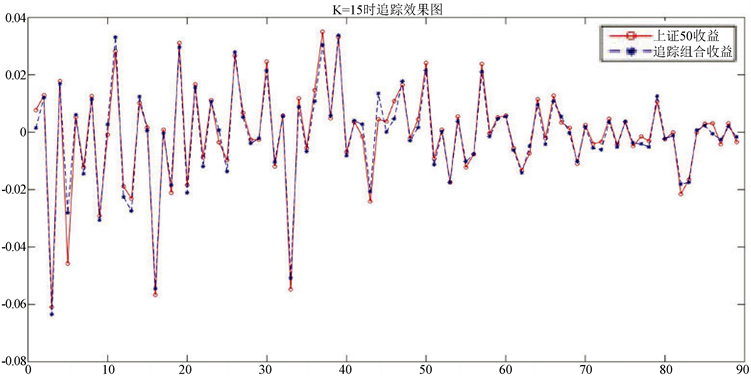Figure 1. Maximum weight picking method in the first case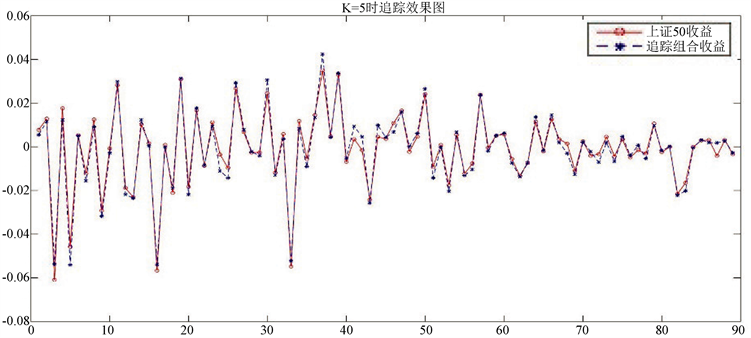Figure 2. Maximum correlation coefficient picking method in the first case

a) 最大权重选股法在K = 15时的追踪组合为：(6.1)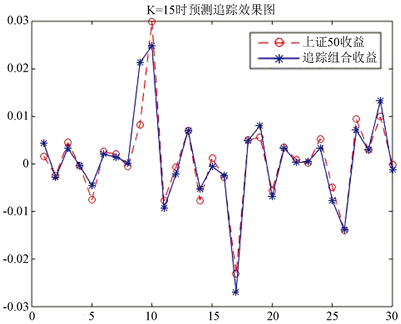Figure 3. Prediction of the maximum weight stock picking method in the first case

b) 最大相关系数选股法在K = 5时的追踪组合为：

$\begin{array}{c}{y}_{t}=0.19268375\ast 招商证券+0.21163009\ast 中国平安+0.16943079\ast 华夏银行\\ \text{\hspace{0.17em}}\text{\hspace{0.17em}}+0.20324995\ast 中国建设+0.22300542\ast 工商银行\end{array}$ (6.2)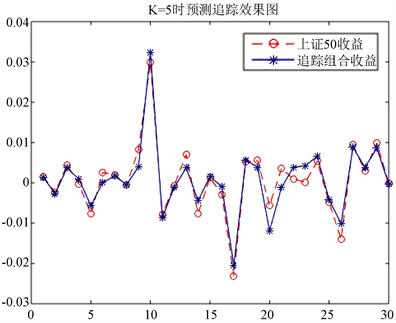Figure 4. Prediction of the maximum coefficient stock selection method in the first case

2) ${\lambda }_{1}=0.5$${\lambda }_{2}=0.5$ 。与第①情况的解答过程相似，得到不同选股方法在训练集中的结果，具体如表3Table 3. Tracking error tables for different stock selections in the training set (2)Table 4. Table of coefficient values for different stock selections in the training set (2)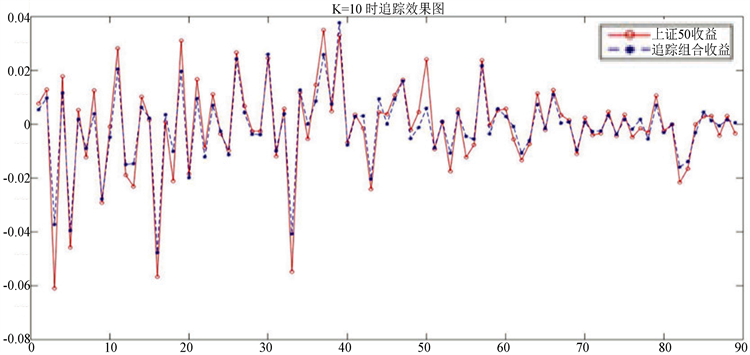Figure 5. Maximum market value selection in the second case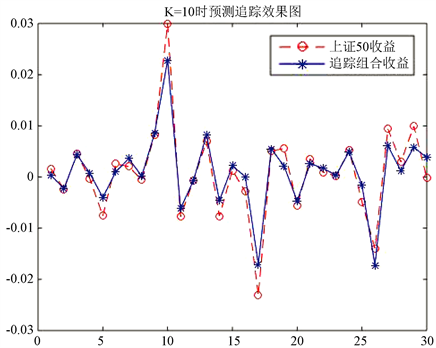Figure 6. Maximum correlation coefficient stock selection method in the second case

a) 最大市值选股法在K = 10时的追踪组合为：(6.3)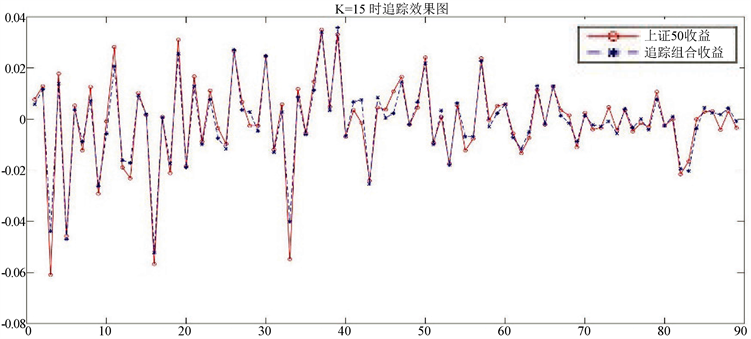Figure 7. Forecast of the largest market value stock picking method in the second case

b) 最大相关系数选股法在K = 15时的追踪组合为：

$\begin{array}{c}{y}_{t}=0.02787847\ast 招商证券+0.05836566\ast 中国平安+0.05093911\ast 华夏银行\\ \text{\hspace{0.17em}}\text{\hspace{0.17em}}+0.03419192\ast 中国建设+0.44478759\ast 工商银行+0.02772875\ast 中信证券\\ \text{\hspace{0.17em}}\text{\hspace{0.17em}}+0.02798614\ast 方正证券+0.02621516\ast 国金证券+0.08790818\ast 兴业银行\\ \text{\hspace{0.17em}}\text{\hspace{0.17em}}+0.06640021\ast 光大银行+0.02996609\ast 中国人寿+0.03408309\ast 中国太保\\ \text{\hspace{0.17em}}\text{\hspace{0.17em}}+0.02776262\ast 海螺水泥+0.02672472\ast 华泰证券+0.02906230\ast 国泰君安\end{array}$ (6.4)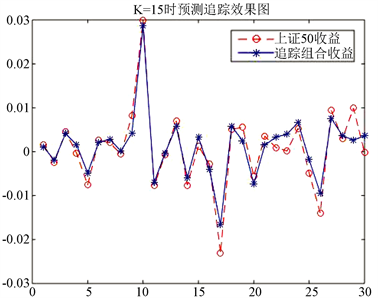Figure 8. Prediction of the maximum correlation coefficient stock picking method in the second case

3) ${\lambda }_{1}=0$${\lambda }_{2}=1$Table 5. Tracking error tables for different stock selections in the training set (3)Table 6. Table of coefficient values for different stock selections in the training set (3)

a) 最大市值选股法在K = 10时的追踪组合为：

$\begin{array}{c}{y}_{t}=0.14196729\ast 工商银行+0.08567349\ast 中国石油+0.11568688\ast 中国银行\\ \text{\hspace{0.17em}}\text{\hspace{0.17em}}+0.13745083\ast 农业银行+0.06920198\ast 中国人寿+0.08546269\ast 中国石化\\ \text{\hspace{0.17em}}\text{\hspace{0.17em}}+0.09877374\ast 中国平安+0.09337528\ast 招商银行+0.10378138\ast 交通银行\\ \text{\hspace{0.17em}}\text{\hspace{0.17em}}+0.06862643\ast 贵州茅台\end{array}$ (6.5)

b) 最大相关系数选股法在K = 10时的追踪组合为：

$\begin{array}{c}{y}_{t}=0.04495734\ast 招商证券+0.09541614\ast 中国平安+0.08110983\ast 华夏银行\\ \text{\hspace{0.17em}}\text{\hspace{0.17em}}+0.05660110\ast 中国建设+0.34684853\ast 工商银行+0.04540740\ast 中信证券\\ \text{\hspace{0.17em}}\text{\hspace{0.17em}}+0.04516149\ast 方正证券+0.04243384\ast 国金证券+0.14232487\ast 兴业银行\\ \text{\hspace{0.17em}}\text{\hspace{0.17em}}+0.09973946\ast 光大银行\end{array}$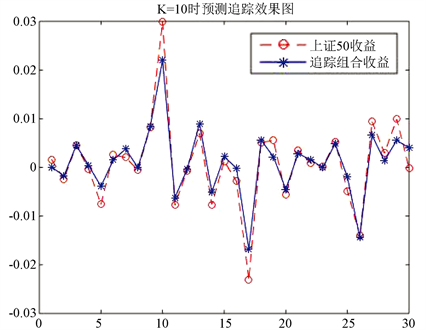Figure 9. Forecast of the largest market value stock picking method in the third caseFigure 10. Prediction of the maximum correlation coefficient stock picking method in the third case

6.2. 基于分位数回归的指数追踪模型的实证分析Table 7. Fitting results of the maximum weight stock selection method in the training set

Table 8. Fitting results of the largest market value picking method in the training set

Table 9. Fitting results of the maximum correlation coefficient stock selection method in the training set

7. 结论

  Markowitz, H. (1952) Portfolio Selection. The Journal of Finance, 7, 77-91.  Gilli, M. and Këllezi, E. (2002) The Threshold Accepting Heuristic for Index Tracking. In: Financial Engineering, E-Commerce and Supply Chain, Springer US, 1-18.  Koenker, R. and Bassett, G. (1978) The Asymptotic Distribution of the Least Absolute Error Estimator. Journal of the American Statistical Association, 73, 618-622. https://doi.org/10.1080/01621459.1978.10480065  Koenker, R. and Bassett Jr., G. (1978) Regression Quantiles. Econometrica: Journal of the Econometric Society, 46, 33-50. https://doi.org/10.2307/1913643  Mezali, H. and Beasley, J.E. (2013) Quantile Regression for Index Tracking and Enhanced Indexation. Journal of the Operational Research Society, 64, 1676-1692. https://doi.org/10.1057/jors.2012.186  Baestaens, D.E. and Bergh, W.M.V. (1994) Neural Network Solutions for Trading in Financial Markets. Pitman Publishing, Inc., London.  Ruiz-Torrubiano, R. and Suárez, A. (2009) A Hybrid Optimization Approach to Index Tracking. Annals of Operations Research, 166, 57-71. https://doi.org/10.1007/s10479-008-0404-4  沈双生, 郭子忠. 指数型基金的发展优势和投资风险分析[J]. 金融教学与研究, 2003(2): 35-36.  范旭东. 跟踪误差与优化指数投资策略——理论分析与实证研究[D]: [硕士学位论文]. 成都: 西南财经大学, 2006.  杨国梁, 赵社涛, 徐成贤. 基于支持向量机的金融市场指数追踪技术研究[J]. 国际金融研究, 2009(10): 68-72.